# 阿里面试官：HashMap 为什么是线程不安全的？

Java 的 HashMap 是非线程安全的。多线程下应该用 ConcurrentHashMap。

• 多线程 put 操作后，get 操作导致死循环。

• 多线程 put 非 NULL 元素后，get 操作得到 NULL 值。

• 多线程 put 操作，导致元素丢失。

### 1、为何出现死循环？（在多线程下使用非线程安全的 HashMap，单线程根本不会出现）

• HashMap 是采用链表解决 Hash 冲突，因为是链表结构，那么就很容易形成闭合的链路，这样在循环的时候只要有线程对这个 HashMap 进行 get 操作就会产生死循环。

• 在单线程情况下，只有一个线程对 HashMap 的数据结构进行操作，是不可能产生闭合的回路的。

• 那就只有在多线程并发的情况下才会出现这种情况，那就是在 put 操作的时候，如果`size>initialCapacity*loadFactor`，那么这时候 HashMap 就会进行 rehash 操作，随之 HashMap 的结构就会发生翻天覆地的变化。很有可能就是在两个线程在这个时候同时触发了 rehash 操作，产生了闭合的回路。

### 2、如何产生的：

```` public V put(K key, V value)`` {``  ......``  //算Hash值``  int hash = hash(key.hashCode());``  int i = indexFor(hash, table.length);``  //如果该key已被插入，则替换掉旧的value （链接操作）``  for (Entry e = table[i]; e != null; e = e.next) {``   Object k;``   if (e.hash == hash && ((k = e.key) == key || key.equals(k))) {``    V oldValue = e.value;``    e.value = value;``    e.recordAccess(this);``    return oldValue;``   }``  }``  modCount++;``  //该key不存在，需要增加一个结点``  addEntry(hash, key, value, i);``  return null;`` }`
```

```` void addEntry(int hash, K key, V value, int bucketIndex)`` {``  Entry e = table[bucketIndex];``  table[bucketIndex] = new Entry(hash, key, value, e);``  //查看当前的size是否超过了我们设定的阈值threshold，如果超过，需要resize``  if (size++ >= threshold)``   resize(2 * table.length);`` }`
```

```` void resize(int newCapacity)`` {``  Entry[] oldTable = table;``  int oldCapacity = oldTable.length;``  ......``  //创建一个新的Hash Table``  Entry[] newTable = new Entry[newCapacity];``  //将Old Hash Table上的数据迁移到New Hash Table上``  transfer(newTable);``  table = newTable;``  threshold = (int)(newCapacity * loadFactor);`` }`
```

```` void transfer(Entry[] newTable)`` {``  Entry[] src = table;``  int newCapacity = newTable.length;``  //下面这段代码的意思是：``  //  从OldTable里摘一个元素出来，然后放到NewTable中``  for (int j = 0; j < src.length; j++) {``   Entry e = src[j];``   if (e != null) {``    src[j] = null;``    do {``     Entry next = e.next;//取出第一个元素``     int i = indexFor(e.hash, newCapacity);``     e.next = newTable[i];``     newTable[i] = e;``     e = next;``    } while (e != null);``   }``  }`` }`
```

### 3、图解 HashMap 死循环：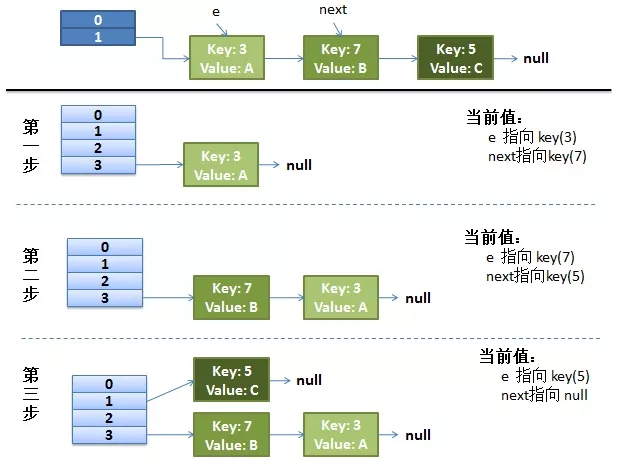1）假设我们有两个线程。

```` do {``  Entry next = e.next; // <--假设线程一执行到这里就被调度挂起了，执行其他操作``  int i = indexFor(e.hash, newCapacity);``  e.next = newTable[i];``  newTable[i] = e;``  e = next;`` } while (e != null);`
```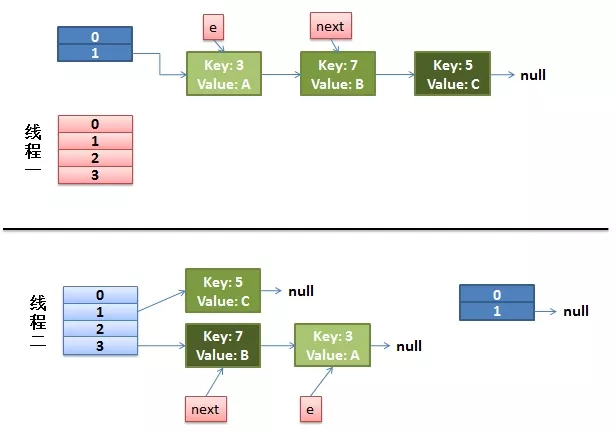2）线程一被调度回来执行。

• 先是执行 `newTalbe[i] = e;`

• 然后是`e = next`，导致了 e 指向了`key(7)`

• 而下一次循环的`next = e.next`导致了 next 指向了`key(3)`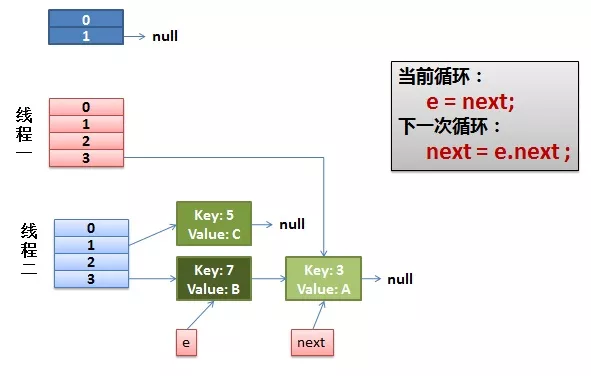3）一切安好。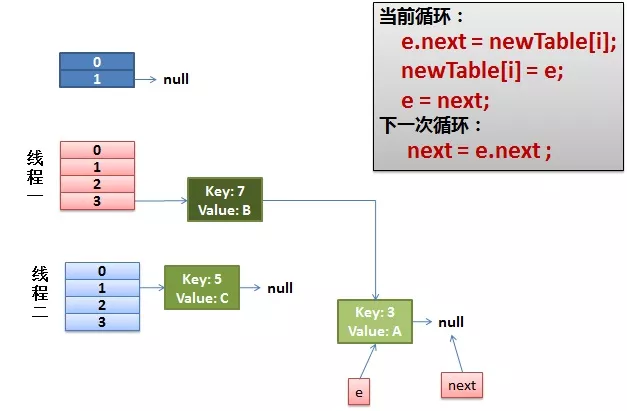4）环形链接出现。

`e.next = newTable[i]` 导致  `key(3).next` 指向了 `key(7)`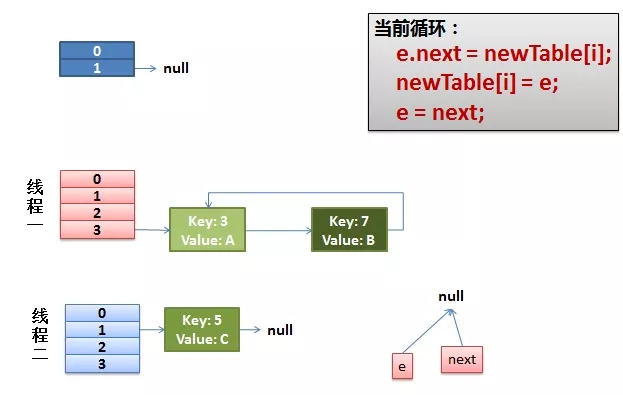...全文
84 1 收藏 回复4.7w+

Java相关技术讨论